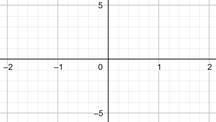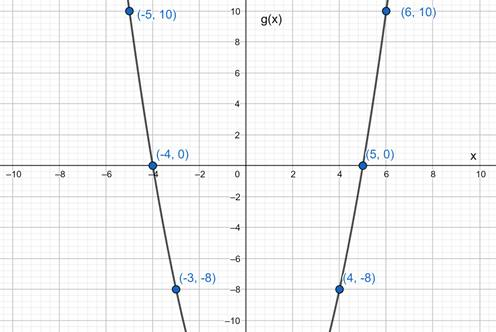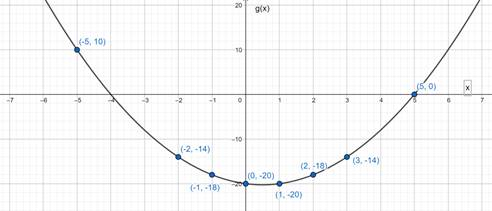# To graph : The function in a specified viewing rectangle.### Precalculus: Mathematics for Calcu...

6th Edition
Stewart + 5 others
Publisher: Cengage Learning
ISBN: 9780840068071### Precalculus: Mathematics for Calcu...

6th Edition
Stewart + 5 others
Publisher: Cengage Learning
ISBN: 9780840068071

#### Solutions

Chapter 2.2, Problem 30E

a.

To determine

## To graph : The function in a specified viewing rectangle.

Expert Solution

### Explanation of Solution

Given information :

g(x)=x2x20

The viewing rectangle is [2,2] by [5,5]

Graph :

 x 0 1 2 −1 −2 g(x) −20 −20 −18 −18 −14There is no value of g(x) in the specified rectangle view.

Interpretation : There is no value of g(x) in the viewing rectangle of [2,2] by [5,5] .

b.

To determine

Expert Solution

### Explanation of Solution

Given information :

g(x)=x2x20

The viewing rectangle is [10,10] by [10,10]

Graph :

 x 4 5 6 −3 −4 −5 g(x) −8 0 10 −8 0 10This is the graph of given function represented in the viewing rectangle of [10,10] by [10,10].

Interpretation : The graph of the given function is represented in the viewing rectangle [10,10] by [10,10]. This graph shows the more appropriate view of the function than the graph shown in the viewing rectangle [2,2] by [5,5] .

c.

To determine

Expert Solution

### Explanation of Solution

Given information :

g(x)=x2x20

The viewing rectangle is [7,7] by [25,20]

Graph :

 x 0 1 2 3 5 −1 −2 −5 g(x) −20 −20 −18 −14 0 −18 −14 10This is the graph of given function represented in the viewing rectangle of [7,7] by [25,20]

Interpretation : The graph of the given function is represented in the viewing rectangle [7,7] by [25,20] . This graph shows the more appropriate view of the function than the graph shown in the viewing rectangle of [10,10] by [10,10] .

d.

To determine

Expert Solution

### Explanation of Solution

Given information :

g(x)=x2x20

The viewing rectangle is [10,10] by [100,100]

Graph :

 x 0 1 3 5 9 −1 −5 −9 g(x) −20 −20 −14 0 52 −18 10 70This is the graph of given function represented in the viewing rectangle of [10,10] by [100,100]

Interpretation : The graph of the given function is represented in the viewing rectangle [10,10] by [100,100] . The graph of the given function is represented appropriately in viewing rectangle of [7,7] by [25,20] and [10,10] by [100,100] . The graph in the viewing rectangle [10,10] by [100,100] gives more complete view among two.

### Have a homework question?

Subscribe to bartleby learn! Ask subject matter experts 30 homework questions each month. Plus, you’ll have access to millions of step-by-step textbook answers!